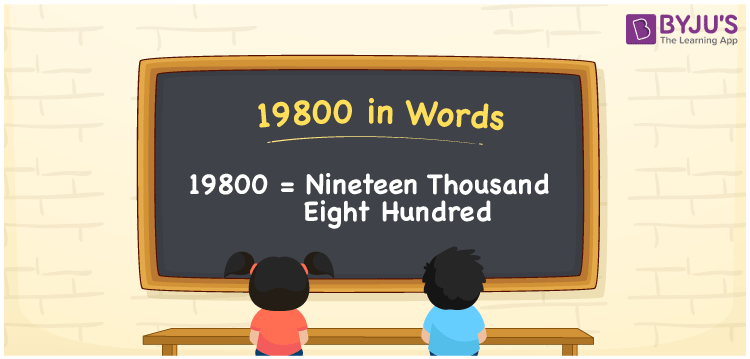# 19800 in Words

We can write 19800 in words as Nineteen Thousand Eight Hundred. It is possible to write the number name for 19800 with the help of a place value chart. It is very easy to write one, two, three-digit numbers in words but to write 5 digit numbers need logical thinking. 19800 is a cardinal number as it denotes a specific amount. Let us learn to write 19800 in words along with FAQs in this article.

 19800 in words Nineteen Thousand Eight Hundred Nineteen Thousand Eight Hundred in numerical form 19800

## 19800 in English Words

Generally, we write numbers in words using the English alphabet. Thus, 19800 in words is spelt as Nineteen Thousand Eight Hundred.## How to Write 19800 in Words?

We know that 19800 is a five-digit number. Hence, we should make a place value chart of up to five digits. Let us learn the conversion of 19800 to words from the below table.

 Ten Thousands Thousands Hundreds Tens Ones 1 9 8 0 0

Therefore, we can write the expanded form as:

1 x Ten Thousand + 9 x Thousand + 8 x Hundred + 0 × Ten + 0 × One

= 1 x 10000 + 9 x 1000 + 8 x 100 + 0 x 10 + 0 x 1

= 10000 + 9000 + 800 + 0 + 0

= 10000 + 9000 + 800

= 19800

= Nineteen Thousand Eight Hundred

Hence, 19800 in words is written as Nineteen Thousand Eight Hundred.

Interesting way of writing 19800 in words

1 = One

19 = Nineteen

198= One Hundred and Ninety-Eight

1980 = One Thousand Nine Eighty

19800 = Nineteen Thousand Eight Hundred

Thus, the word form of the number 19800 is Nineteen Thousand Eight Hundred.

19800 is a natural number that precedes 19801 and succeeds 19799

• 19800 in words – Nineteen Thousand Eight Hundred
• Is 19800 an odd number? – No
• Is 19800 an even number? – Yes
• Is 19800 a perfect square number? – No
• Is 19800 a perfect cube number? – No
• Is 19800 a prime number? – No
• Is 19800 a composite number? – Yes

## Frequently Asked Questions on 19800 in Words

Q1

### How do you express 19800 in words?

We can express 19800 in words as Nineteen Thousand Eight Hundred.
Q2

### Find the value of 17410 + 2390 in words.

17410 + 2390 = 19800 Hence, the value of 17410 + 2390 in words is Nineteen Thousand Eight Hundred.
Q3

### 19800 is a composite number. True or False.

True, 19800 is a composite number.